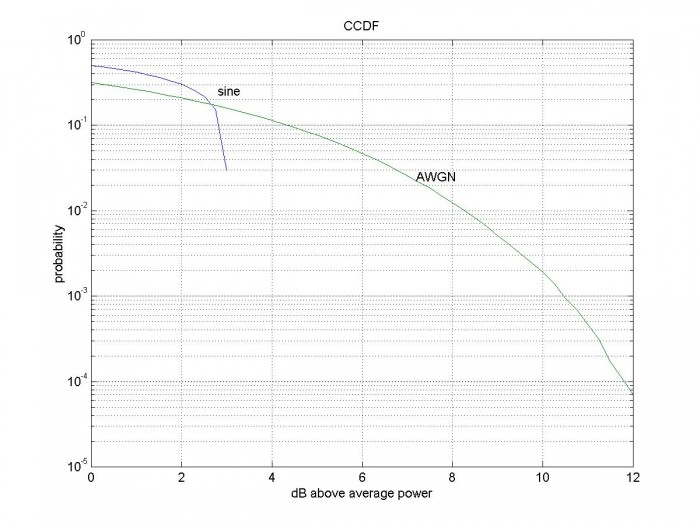# Peak to Average Power Ratio and CCDF

Peak to Average Power Ratio (PAPR) is often used to characterize digitally modulated signals.  One example application is setting the level of the signal in a digital modulator.  Knowing PAPR allows setting the average power to a level that is just low enough to minimize clipping.

However, for a random signal, PAPR is a statistical quantity.  We have to ask, what is the probability of a given peak power?  Then we can decide where to set the average power level such that clipping occurs at an acceptably low rate.

Let Pratio = the ratio of the instantaneous power of a sample to the average power of the signal.  The probability that Pratio exceeds a given value is called the Complementary Cumulative Distribution Function (CCDF).  Given a signal vector x, the CCDF y is computed as follows:

```papr = 0:.25:12;         % dB vector of PAPR values
P = x.^2;                % W power of each sample of vector x
Pratio = P/mean(P);      % power/average power
PdB = 10*log10(Pratio);
for i = 1:length(papr)
y(i) = length(find(PdB >= papr(i)))/N;     % # of samples exceeding papr(i)
end
```
In the attached m-file, we calculate the CCDF for a sine wave and for Gaussian noise.  Since the sine wave is periodic, we need only use a single period.  For the noise, we need a lot of samples to find the low probability amplitudes:  using 100,000 samples yields 7 samples with 10log10(Pratio) > 12 dB.

The figure below shows CCDF for a sine wave and for 100,000 samples of Gaussian noise.  The PAPR of the sine wave is the maximum value vs. the x-axis.  This is of course 20*log10[Vpeak/Vrms] = 20*log10[sqrt(2)] = 3.01 dB.CCDF of a sine wave and CCDF of AWGN (100,000 samples).

## References

“Characterizing Digitally Modulated Signals with CCDF Curves”, Agilent Technologies, 2000.
http://cp.literature.agilent.com/litweb/pdf/5968-6...

```%PAPR_demo.m 4/1/16 nr
% PAPR = peak to average power ratio
% Complimentary Cumulative Distribution Function (CCDF) of real signal x.
% x     real signal vector
% papr  vector of papr values
% y     CCDF of x vs. papr
papr = 0:.25:12; % dB vector of PAPR values
% PAPR of sine wave
f = 1; % Hz sine frequency
Ts = 1/1000;                 % s sample time
N= 1000;                     % number of samples
n= 0:N-1;                    % time index
t= n*Ts;                     % s
x = sin(2*pi*f*t);           % signal = one cycle of a sine wave
P = x.^2;                    % W power of each sample of vector x
Pratio = P/mean(P);          % power/average power
PdB = 10*log10(Pratio);
for i = 1:length(papr)
y(i) = length(find(PdB >= papr(i)))/N;     % # of samples exceeding papr(i)
end
% PAPR of AWGN
randn('state',0);                     % reset random number generator
M = 100000; % number of samples
x_gauss = randn(M,1);                 % signal = AWGN
Pratio = x_gauss.^2;                  % power/average power (average power = 1)
PdB = 10*log10(Pratio);
for i = 1:length(papr)
y_gauss(i) = length(find(PdB > papr(i)))/M;
end
semilogy(papr,y,papr,y_gauss),grid
xlabel('dB above average power'),ylabel('probability')
title('CCDF'),axis([0 12 1e-5 1])
text(2.8,.25,'sine'),text(7.2,.025,'AWGN')
```
5/14/16    revised 6/25/22

[ - ]
Comment by May 29, 2016interesting! you can format code in a fixed width font by surrounding with < code > < / code > blocks
[ - ]
Comment by May 29, 2016Thanks.
[ - ]
Comment by March 4, 2022Very helpful Neil. Where is the "give a beer" button, I would like to pass one to you!

[ - ]
Comment by March 4, 2022Thanks.  Nice to see an old post get noticed!

Neil

To post reply to a comment, click on the 'reply' button attached to each comment. To post a new comment (not a reply to a comment) check out the 'Write a Comment' tab at the top of the comments.